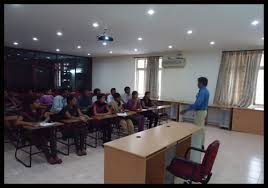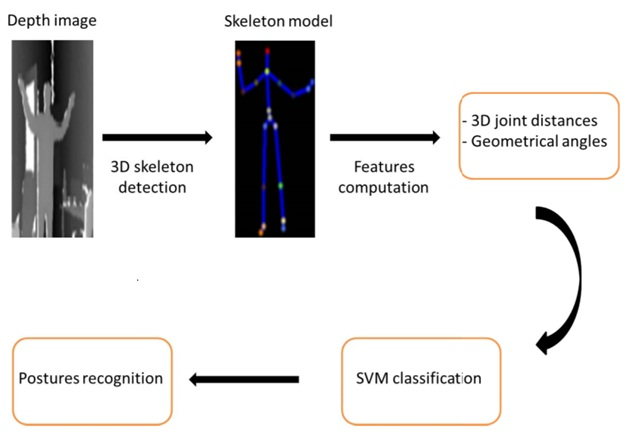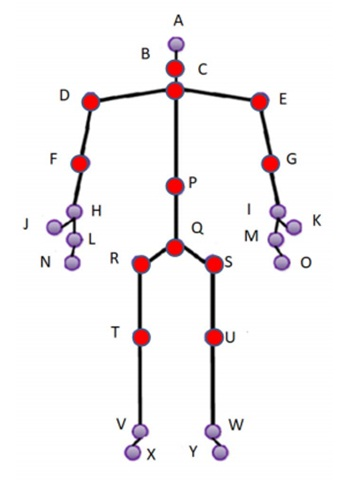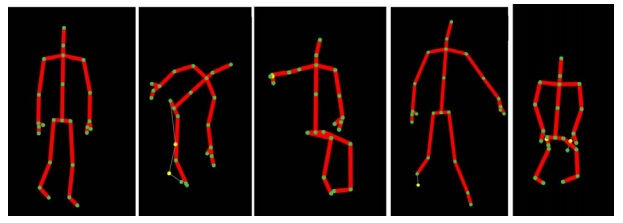## Human Posture Recognition System

Published on Jan 03, 2023

### Abstract

This paper presents a human posture recognition system based on depth imaging. The proposed system is able to efficiently model human postures by exploiting the depth information captured by an RGB-D camera. Firstly, a skeleton model is used to represent the current pose. Human skeleton configuration is then analyzed in the 3D space to compute joint-based features.

Our feature set characterizes the spatial configuration of the body through the 3D joint pairwise distances and the geometrical angles defined by the body segments. Posture recognition is then performed through a supervised classification method. To evaluate the proposed system we created a new challenging dataset with a significant variability regarding the participants and the acquisition conditions. The experimental results demonstrated the high precision of our method in recognizing human postures, while being invariant to several perturbation factors, such as scale and orientation change. Moreover, our system is able to operate efficiently, regardless illumination conditions in an indoor environment, as it is based depth imaging using the infrared sensor of an RGB-D camera. Index Terms—RGB-D camera, depth imaging, posture recognition, SVM.

### The Proposed Method

Our system uses a single RGB-D camera (Microsoft Kinect v2) to capture depth maps and build a body skeleton. We then use the 3D joint positions to compute posture features including

(1) the 3D pairwise joint distances and

(2) the geometrical angles defined by adjacent body segments.

A supervised classification method is finally applied to recognize statics human postures. In summary, the system consists of three main modules (see figure 1): skeleton estimation, feature computation, and postures classification.### 3D Skeleton Estimation

To build the 3D model of human posture, we use the skeleton detection method  to localize different body parts. This method is quite accurate in modeling the human body as an articulated structure of connected segments. This can be achieved in real-time by the only use of depth images. The method of Shotton et al. uses  a random forest classifier to segment the different human body parts through a pixellevel classification from single depth images. A mean shift mode detection is then applied to find local centroids of the body part probability mass and generate the 3D locations of body joints. The obtained 3D skeleton formed by 25 joints represents the human posture as illustrated in the figure 2.

###Figure 2.The 3D joints defining the skeleton. Joints corresponding to the considered geometrical angles are represented in red.

### Computing Features

Constructing a robust feature set is a crucial step in posture recognition systems. In our work, we used 2 types of features to represent human postures: the 3D pairwise joint distances and the geometrical angles defined by adjacent segments.

Considering the set of N = 25 joints (shown in figure 2), the 3D joint coordinates are extracted as:

X = {Ji = (xi, yi, zi), i = 1, 2, ..., N} (1)

where, Ji is the joint number i obtained from the body skeleton and the triplet (xi, yi, zi) represents the 3D coordinates of Ji. Once joint positions are extracted, we compute 2 types of features representing human body posture: 1) the 3D pairwise distances between joints, and 2) geometrical angles of adjacent segments.

1.3D joint distances:Given the body skeleton obtained from depth images, we calculate the relative 3D distances between pairs of joints. Such features were successfully used to model human body for action recognition . We calculate the feature vector as:

D = {dist(Ji, Jj )|i, j = 1, 2, ..., N;i = j} (2)

wheredist(Ji, Jj ) is the Euclidean distance between two joints Ji and Jj . To ensure scale invariance and to remove the impact of body height variation, the 3D distances are normalized with respect to the person’s height. The normalization process is performed by considering the distance BP (the 3D distance between the spine shoulder joint and the spine middle joint). The choice of this segment is based on experimental observations concluding that the distance BP is sufficiently stable with respect to several body deformations, in addition to its proportionality to the person’s height.

### Geometrical Angles

In addition to 3D joint distances, our joint-based features include geometrical angles corresponding to relevant joints. Based on our dataset, we selected experimentally a subset of joints according to their importance in characterizing the studied human postures. In the figure 2, the selected joints are represented in red. We considered m = 12 angles as follows: (CB, CP ), (CP, CD ), (CE, CP ), (DC, DF ),(EC, EG ), (DR, DF ), (ES, EG ), (PC, P Q ), (QT, QU ), (RT, RQ ), (SQ, SU ) and (QR , QS ).

Geometric angles defined by adjacent segments are directly estimated from joint positions. We define the geometrical angle feature vector as:

A = {θk(u, v)|k = 1, 2, ..., K}-----------------(3)

The angle θk between the two adjacent segments u and v is calculated using the 3D coordinates as:

θk(u, v) = arccosu.v /||u|| .|| v||----------------(4)

The two feature sets D and A are then concatenated in a single feature vector to form the final representation of human posture F = [D, A].

### Posture Classification

Once the body pose is modeled, posture recognition is performed in a supervised classification approach using SVM classifier. With its different kind of kernels, SVM has the ability to generate non-linearly as well as high-dimensional classification issue. The SVM classifier also supports multipleclass problems by computing the hyperplane between each class and the rest. In our work, we used an SVM with a linear kernel, since it outperformed other classifiers as discussed in the next section.

### Experiments

Datasets: We created a new dataset that we named KSRD (Kinect Posture Recognition Dataset) using the Microsoft Kinect sensor v2 1. To the best of our knowledge, this is the first dataset created with the second version of the Kinect sensor (using Time of flight technology for depth sensing). Note that a recent study demonstrated that the second version of the Kinect sensor surpasses Kinect v1 significantly in terms of accuracy and precision . In our experimental setup, the camera is mounted on a tripod placed in a corner of our laboratory.

We consider 5 classes of postures: standing, bending, sitting, walking, and crouching. Each posture was performed by 10 participants of different ages, gender, and morphological characteristics. In order to increase the variability of our dataset and evaluate our system in handling scale and orientation change, each posture is captured at 4 different orientations and 4 different distances with respect to the camera. The distance varies from 1 meter to 4 meters while the orientation angle is changing between 0 and 360 degrees. Our dataset includes a total number of 800 observations that we used for training and testing the proposed system. The dataset is randomly divided into training and testing set using the K-Fold Cross-Validation method (with K = 10).

Figure 3 shows examples of human body skeletons corresponding to the 5 classes## Results and Discussion

We designed our experiments in order to analyze the performance of our method according to several aspects. Our first experiment set aimed to identify the optimal choices regarding the two main system components:

1) the feature set and

2) the classification method.

We thus investigated several choices implying 5 classifiers (linear discriminant, quadratic discriminant, linear SVM, quadratic SVM, and cubic SVM) and 3 feature sets (geometrical angles, pairwise joint distances, and the combination of the 2 types of features). Table I summarizes accuracy results for the tested classifiers applied to different feature sets. We can remark that the best recognition rates of linear discriminant classifiers were obtained by using the geometrical angles as features. However, SVM classifiers clearly outperformed linear discriminant methods regardless the feature set. This investigation shows that the linear SVM applied to the entire feature set achieves the best recognition rate (88.3%). We therefore base our recognition system on

1) linear SVM classification, and

2) a combination of the 2 types of features.

One of the major difficulties of pose recognition is the scale change. Our method is designed to handle this problem through the normalization of 3D distance features. On the other hand, our dataset includes participants of different heights, whose poses are captured at 4 different distances ranging from 1 to 4 meters from the camera. In order to evaluate the scale invariance of the proposed system, we carried out another sequence of tests where we trained 4 linear SVM classifiers. Each classifier is trained using poses captured at 3 distances (by excluding 1 subset corresponding to 1 distance in each training procedure). The tests are then performed on the excluded subsets. Table IV shows that the proposed method is scale invariant, as it is able to achieve high recognition rates regardless the distance to the camera. In these experiments, the system performance was stable for the 4 tested distances, with a recognition rate greater than (81%). Note that the highest recognition rate was achieved at about 2 meters. This is consistent with the experimental evaluation of the Kinect v2 , showing that depth accuracy is optimal at this distance. To sum up, our method allowed to achieve high accuracy, while being flexible and invariant to several perturbation factors

### Conclusion

We presented a novel method for human posture recognition using an RGB-D camera. Our method includes 3 main steps: skeleton estimation, feature computation, and posture classification. Firstly, a 3D skeleton is estimated from depth images. Joint-based features are then computed for modeling the observed human pose. Posture classification is finally carried out using a linear SVM. Our method was validated on a challenging dataset released in our laboratory. The proposed system demonstrated high recognition rates and a significant invariance to important perturbation factors, including scale, orientation, and illumination changes. Our future work will focus on developing appropriate mechanisms addressing other difficulties, such as partial occlusion and fast body movements. This also includes extending the dataset by considering these challenging conditions.

### REFERENCES

1. B. Boulay, F. Bremond, and M. Thonnat, Human posture recognition in video sequence. IEEE International Workshop on VS-PETS, Visual Surveillance and Performance Evaluation of Tracking and Surveillance, 2003.

2. R. Gouiaa and J. Meunier, Human posture recognition by combining silhouette and infrared cast shadows. In Image Processing Theory, Tools and Applications (IPTA), 2015 International Conference on, proceedings, 2015.

3. R. Gouiaa and J. Meunier, Human posture classification based on 3D body shape recovered using silhouette and infrared cast shadows. In Image Processing Theory, Tools and Applications (IPTA), 2015 International Conference on, proceedings, 2015.

4. T. Moeslund and E. Granum, 3D human pose estimation using 2D-data and an alternative phase space representation. Procedure Humans, 2000.

5. A. Agarwal and B. Triggs, Recovering 3D human pose from monocular images. IEEE transactions on pattern analysis and machine intelligence, 28(1): 44–58, 2006.

6. A. Fossati, M. Dimitrijevic, V. Lepetit, and P. Fua, From canonical poses to 3D motion capture using a single camera. IEEE transactions on pattern analysis and machine intelligence, 32(7): 1165–1181, 2010.